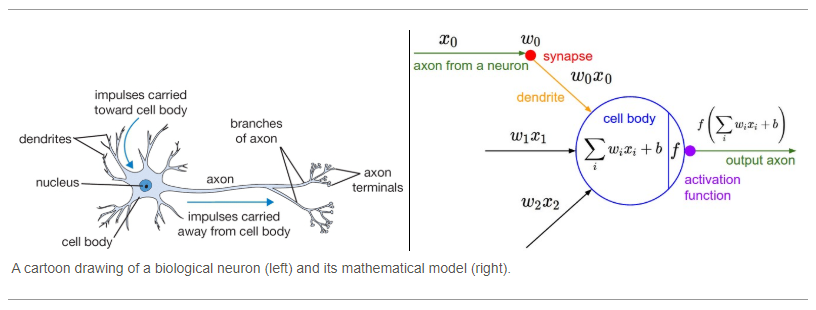# Everything you need to know about “Activation Functions” in Deep learning models

Source: Deep Learning on Medium# Contents

1. What is an activation function and what does it do in a network?
2. Why is there a need for it and why not use a linear function instead?
3. What are the desirable features in an activation function?
4. Various non-linear activations in use
5. Notable non-linear activations coming out of latest research
6. How (and which) to use them in deep neural networks

# What is an activation function?

Simply put, an activation function is a function that is added into an artificial neural network in order to help the network learn complex patterns in the data. When comparing with a neuron-based model that is in our brains, the activation function is at the end deciding what is to be fired to the next neuron. That is exactly what an activation function does in an ANN as well. It takes in the output signal from the previous cell and converts it into some form that can be taken as input to the next cell. The comparison can be summarized in the figure below.

# Why is there a need for it?

There are multiple reasons for having non-linear activation functions in a network.

1. Apart from the biological similarity that was discussed earlier, they also help in keeping the value of the output from the neuron restricted to a certain limit as per our requirement. This is important because input into the activation function is W*x + b where W is the weights of the cell and the x is the inputs and then there is the bias b added to that. This value if not restricted to a certain limit can go very high in magnitude especially in case of very deep neural networks that have millions of parameters. This will lead to computational issues. For example, there are some activation functions (like softmax) that out specific values for different values of input (0 or 1).
2. The most important feature in an activation function is its ability to add non-linearity into a neural network. To understand this, let’s consider multidimensional data such as shown in the figure below: